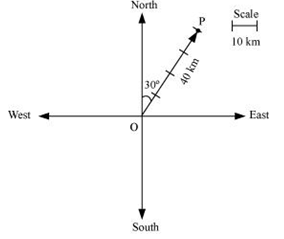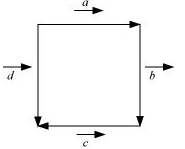JEE  >  NCERT Solutions: Exercise - 10.1 - Vector Algebra

# Exercise - 10.1 - Vector Algebra NCERT Solutions - Mathematics (Maths) Class 12 - JEE

Question 1: Represent graphically a displacement of 40 km, 30° east of north.Here, vector  represents the displacement of 40 km, 30° East of North.

Question 2: Classify the following measures as scalars and vectors.

(i) 10 kg

(ii) 2 metres north-west

(iii) 40°

(iv) 40 watt

(v) 10–19 coulomb

(vi) 20 m/s2

ANSWER : - (i) 10 kg is a scalar quantity because it involves only magnitude.

(ii) 2 meters north-west is a vector quantity as it involves both magnitude and direction.

(iii) 40° is a scalar quantity as it involves only magnitude.

(iv) 40 watts is a scalar quantity as it involves only magnitude.

(v) 10–19 coulomb is a scalar quantity as it involves only magnitude.

(vi) 20 m/s2 is a vector quantity as it involves magnitude as well as direction.

Question 3: Classify the following as scalar and vector quantities.

(i) time period

(ii) distance

(iii) force

(iv) velocity

(v) work done

ANSWER : - (i) Time period is a scalar quantity as it involves only magnitude.

(ii) Distance is a scalar quantity as it involves only magnitude.

(iii) Force is a vector quantity as it involves both magnitude and direction.

(iv) Velocity is a vector quantity as it involves both magnitude as well as direction.

(v) Work done is a scalar quantity as it involves only magnitude.

Question 4: In Figure, identify the following vectors.ANSWER : - (i) Vectors  a and  d are coinitial because they have the same initial point.

(ii) Vectors b and d are equal because they have the same magnitude and direction.

(iii) Vectors a and  c are collinear but not equal. This is because although they are parallel, their directions are not the same.

Question 5: Answer the following as true or false.

(i) a and b are collinear.

(ii) Two collinear vectors are always equal in magnitude.

(iii) Two vectors having same magnitude are collinear.

(iv) Two collinear vectors having the same magnitude are equal.

Vectors  a and b are parallel to the same line.

(ii) False.

Collinear vectors are those vectors that are parallel to the same line.

(iii) False.

It is not necessary for two vectors having the same magnitude to be parallel to the same line.

(iv) False.

Two vectors are said to be equal if they have the same magnitude and direction, regardless of the positions of their initial points.

The document Exercise - 10.1 - Vector Algebra NCERT Solutions | Mathematics (Maths) Class 12 - JEE is a part of the JEE Course Mathematics (Maths) Class 12.
All you need of JEE at this link: JEE

## Mathematics (Maths) Class 12

206 videos|264 docs|139 tests

## FAQs on Exercise - 10.1 - Vector Algebra NCERT Solutions - Mathematics (Maths) Class 12 - JEE

 1. What is vector algebra?Ans. Vector algebra is a branch of mathematics that deals with the manipulation and analysis of vectors, which are quantities that have both magnitude and direction. It involves operations such as addition, subtraction, scalar multiplication, dot product, and cross product.
 2. How is vector addition done?Ans. Vector addition is performed by adding the corresponding components of two or more vectors. For example, if we have two vectors A and B with components (Ax, Ay, Az) and (Bx, By, Bz) respectively, the sum of the vectors A and B is given by (Ax + Bx, Ay + By, Az + Bz).
 3. What is a scalar multiple of a vector?Ans. A scalar multiple of a vector is obtained by multiplying the magnitude of the vector by a scalar quantity. This operation does not change the direction of the vector, only its magnitude. For example, if we have a vector A with components (Ax, Ay, Az) and we multiply it by a scalar quantity k, the result is (kAx, kAy, kAz).
 4. What is the dot product of two vectors?Ans. The dot product of two vectors A and B is a scalar quantity calculated by taking the sum of the products of their corresponding components. Mathematically, it is given by A · B = Ax * Bx + Ay * By + Az * Bz. The dot product is used to calculate the angle between two vectors and has various applications in physics and engineering.
 5. How is the cross product of two vectors calculated?Ans. The cross product of two vectors A and B is a vector quantity that is perpendicular to both A and B. Its magnitude is given by |A × B| = |A| |B| sin(θ), where |A| and |B| are the magnitudes of the vectors and θ is the angle between them. The direction of the cross product is determined by the right-hand rule.

## Mathematics (Maths) Class 12

206 videos|264 docs|139 testsExplore Courses for JEE examSignup to see your scores go up within 7 days! Learn & Practice with 1000+ FREE Notes, Videos & Tests.
10M+ students study on EduRev
Track your progress, build streaks, highlight & save important lessons and more!
Related Searches

,

,

,

,

,

,

,

,

,

,

,

,

,

,

,

,

,

,

,

,

,

;